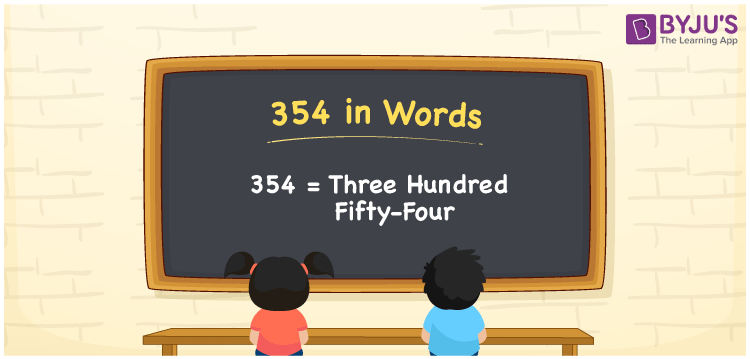# 354 in Words

We can write 354 in words as Three hundred fifty-four. If you purchased a dozen apples, that costs Rs. 354, then you can say, “I purchased a dozen apples at Three hundred fifty-four rupees”. Thus, we generally express or spell numerals using their word forms. In this article, you will learn how to convert the number 354 into words in an easily understandable format.

 354 in words Three hundred fifty-four Three hundred fifty-four in Numbers 354

## 354 in English Words

We generally use the English alphabet to write numbers in words. Thus, we spell 354 in English words as “Three hundred fifty-four”.## How to Write 354 in Words?

The below table shows the place values for all three digits of the number 354. Now, we can obtain the word form of the number 354, as explained below.

 Hundreds Tens Ones 3 5 4

Here, ones = 4, tens = 5, hundreds = 3

Let us expand the above digits with respect to their place values.

3 × Hundred + 5 × Ten + 4 × One

= 3 × 100 + 5 × 10 + 4 × 1

= 300 + 50 + 4

= Three hundred + Fifty + Four

= Three hundred fifty-four

Therefore, 354 in words is written as Three hundred fifty-four.

354 is a natural number that precedes 355 and succeeds 353.

354 in words – Three hundred fifty-four

Is 354 an even number? – Yes

Is 354 an odd number? – No

Is 354 a prime number? – No

Is 354 a composite number? – Yes

Is 354 a perfect square number? – No

Is 354 a perfect cube number? – No

## Frequently Asked Questions on 354 in Words

Q1

### How do you write 354 in words?

We can write the number 354 in English words as Three hundred fifty-four.
Q2

### Write the number 354.354 in word form.

The word form of the decimal number 354.354 is “Three hundred fifty-four point three five four”.
Q3

### How to write Rs. 354 in words on a cheque?

On a cheque, we can express an amount of Rs. 354 in words as “Three hundred fifty-four rupees only”.